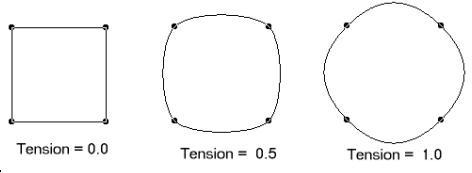﻿ Tension Property - Namespace: Leadtools.Annotations.Engine - Assembly: Leadtools.Annotations.Engine - Raster, Medical, Document Help
←Select platform
In This Topic ▼

# Tension Property

Summary

Gets or sets a value that specifies the amount that this AnnCurveObject curve bends between control points.

Syntax
C#
VB
Java
Objective-C
C++
``public double Tension { get; set; } ``
``Public Property Tension As Double ``
``@property (nonatomic) double tension; ``
````public double getTension() `
`public void setTension(double tension) ````
````public:  `
`   property double Tension `
`   { `
`      double get() `
`      void set(double value) `
`   } ````

#### Property Value

A value that specifies the amount that this AnnCurveObject curve bends between control points.

Remarks

Valid values are between 0.0 and 1.0, where 0.0 indicates straight lines, and 1.0 indicates lines with the highest curvature. A typical value is 0.5. These values for Tension for a Closed Curve object are shown in the following bitmap:Requirements